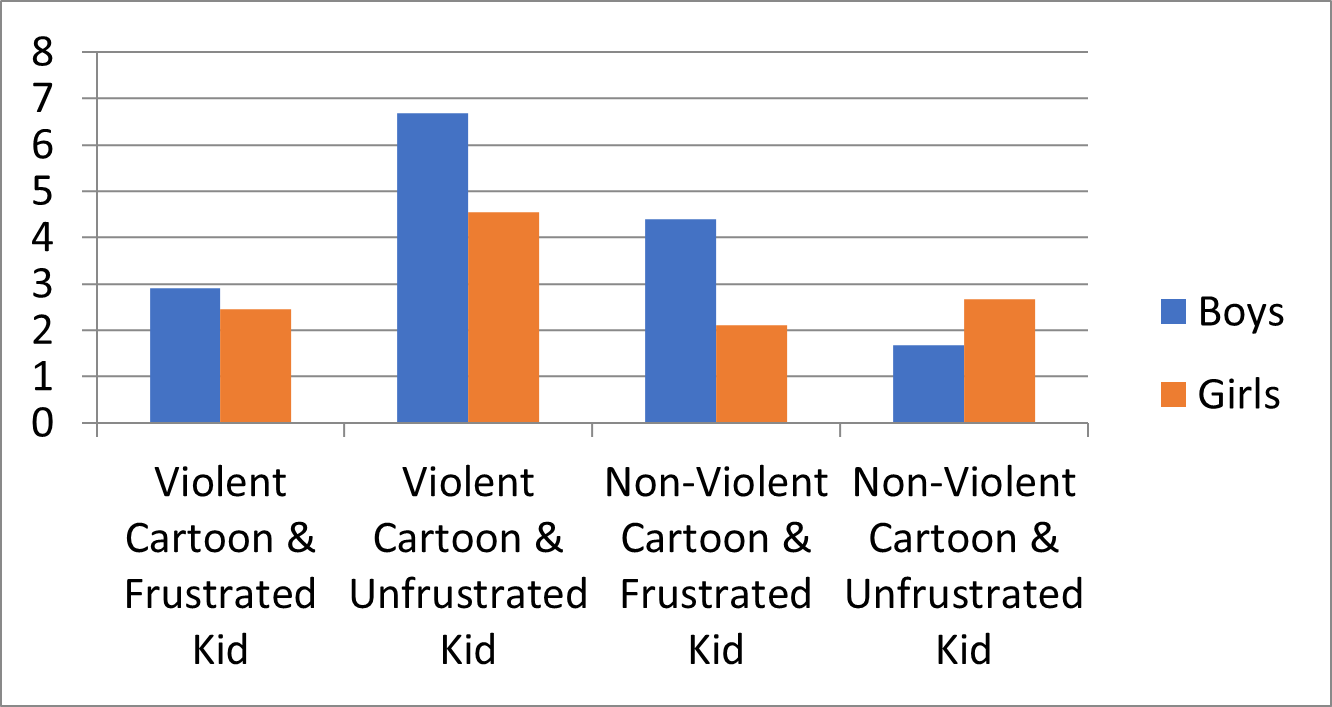# 13.2.2: Graphing Main Effects and Interactions

•• Michelle Oja
• Taft College
$$\newcommand{\vecs}{\overset { \rightharpoonup} {\mathbf{#1}} }$$ $$\newcommand{\vecd}{\overset{-\!-\!\rightharpoonup}{\vphantom{a}\smash {#1}}}$$$$\newcommand{\id}{\mathrm{id}}$$ $$\newcommand{\Span}{\mathrm{span}}$$ $$\newcommand{\kernel}{\mathrm{null}\,}$$ $$\newcommand{\range}{\mathrm{range}\,}$$ $$\newcommand{\RealPart}{\mathrm{Re}}$$ $$\newcommand{\ImaginaryPart}{\mathrm{Im}}$$ $$\newcommand{\Argument}{\mathrm{Arg}}$$ $$\newcommand{\norm}{\| #1 \|}$$ $$\newcommand{\inner}{\langle #1, #2 \rangle}$$ $$\newcommand{\Span}{\mathrm{span}}$$ $$\newcommand{\id}{\mathrm{id}}$$ $$\newcommand{\Span}{\mathrm{span}}$$ $$\newcommand{\kernel}{\mathrm{null}\,}$$ $$\newcommand{\range}{\mathrm{range}\,}$$ $$\newcommand{\RealPart}{\mathrm{Re}}$$ $$\newcommand{\ImaginaryPart}{\mathrm{Im}}$$ $$\newcommand{\Argument}{\mathrm{Arg}}$$ $$\newcommand{\norm}{\| #1 \|}$$ $$\newcommand{\inner}{\langle #1, #2 \rangle}$$ $$\newcommand{\Span}{\mathrm{span}}$$$$\newcommand{\AA}{\unicode[.8,0]{x212B}}$$

Let's back to our example on distraction and use the following graphs to help interpret the main effects of reward, the main effects of distraction, and to see how they interact.

## Distraction Effect:  What Do You See?

Results from 2x2 designs are also often plotted with line graphs. Figure $$\PageIndex{1}$$ shows four different graphs, using bars and lines to plot the same means. Dr. Crump wanted to show you this so that you realize that how you graph your data matters, and it makes it more or less easy for people to understand the results. Also, how the data is plotted matters for what you need to look at to interpret the results.Figure $$\PageIndex{1}$$: The same example means plotted using bar graphs or line graphs, and with Distraction or Reward on the x-axis.  (CC-BY-SA Matthew J. C. Crump from Answering Questions with Data- Introductory Statistics for Psychology Students)

What do you see in both of the graphs on the left?  Dr. MO sees that distraction matters, but distraction is particularly effective in reducing the number of differences spotted when there is no reward.  In both graphs on the left of Figure $$\PageIndex{1}$$, the one really low bar or dot is when there is no reward and the participant was distracted.

What do you see in the graphs on the right in Figure $$\PageIndex{1}$$?  The first thing that Dr. MO saw was that the line chart makes it clear that there's a high level of spotting differences in the No Distraction condition.  Then she noticed that the dot for participants who were Distracted and Not Rewarded was way low compared to all of the other dots.  The bar chart shows that as well; the left most bar is clearly shorter than all of the others.

What you are seeing in these charts are the main effects and interactions.  The main effect of IV1 shows that distraction matters; those participants who were distracted spotted fewer distractions.  The main effect of IV2 shows that reward matters; those participants who were rewarded spotted more distractions.  And finally, these charts show an interaction.  Although both variables mattered, their individual effects seemed to be magnified when combined.  Participants who were Distracted and Not Rewarded were pretty horrible at spotting differences, more horrible than either IV's main effect would suggest on its own.  That's the power of interactions.

## On the Playground:  What Do You See?

Let's try another one.  Check out Figure $$\PageIndex{2}$$ and determine the factorial notations.  Figure $$\PageIndex{2}$$ is a bar chart that shows the means of the different conditions (levels of the IV).  This is different from the frequency charts that we first learned about.  When not listed, it can be helpful to list the IVs, so we’ll start with that.  The DV is number of times a student was pushed on the playground.Figure $$\PageIndex{2}$$: Example of Factorial Design in a Bar Chart. (CC-BY-SA; graph created by Michelle Oja)

Let's answer the questions that we talked about when identifying factorial notations about Figure $$\PageIndex{2}$$ to see if we can find the main effects and interactions.

Exercise $$\PageIndex{3}$$

For Figure $$\PageIndex{2}$$:

1. To determine one main effect, what are the levels of one of the IVs?  What could you name that IV?
2. To determine the other main effect, what are the levels of another of the IVs?  What could you name that IV?
3. There’s one more main effect!  What are the levels of the last IV?  What could you name that IV?

It doesn’t matter what order you found these in:

1. One IV’s level is Boys or Girls, so that could be called the main effect of “Gender”.
2. Another IV’s levels looks like violent or non-violent cartoons.  That main effect could be called "Cartoon" or "Cartoon Type" or "Cartoon Violence."
3. A final IV’s levels is frustrated kid or unfrustrated kid.  We could call that main effect "Mood".

Now that we see the main effects, let’s determine what kind of factorial design is shown in Figure $$\PageIndex{1}$$.

Exercise $$\PageIndex{4}$$

What kind of factorial designs is shown in Figure $$\PageIndex{2}$$?  2x2?  2x3?  2x2x2? Other?

Figure $$\PageIndex{2}$$ shows a 2x2x2